Next: Energy Equation Up: Continuity Equation Previous: Continuity Equation   Contents

## Expression for Momentum Density

It is useful to describe the equation for momentum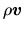. Eulerian derivative of momentum densityis rewritten as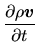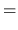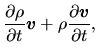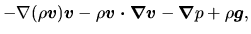(A.12)

where we used equations (A.7) and (A.11). In the Cartesian cordinate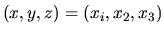, this becomes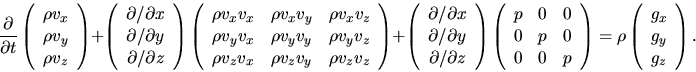(A.13)

Using the momentum stress tensor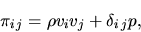(A.14)

the above equation is written as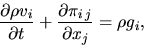(A.15)

where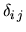represents the Kronecker's delta as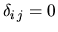for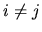and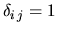for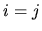.

If there is no external force, rhs of equation (A.15) is equal to zero. If the momentum densitywere to obey the continuity equation, equation (A.15) would be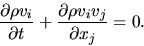(A.16)

However, this is incorrect, because there exists the pressure force in the fluid and thus the momentum of the fluid element is not conserved.Next: Energy Equation Up: Continuity Equation Previous: Continuity Equation   Contents
Kohji Tomisaka 2007-07-08